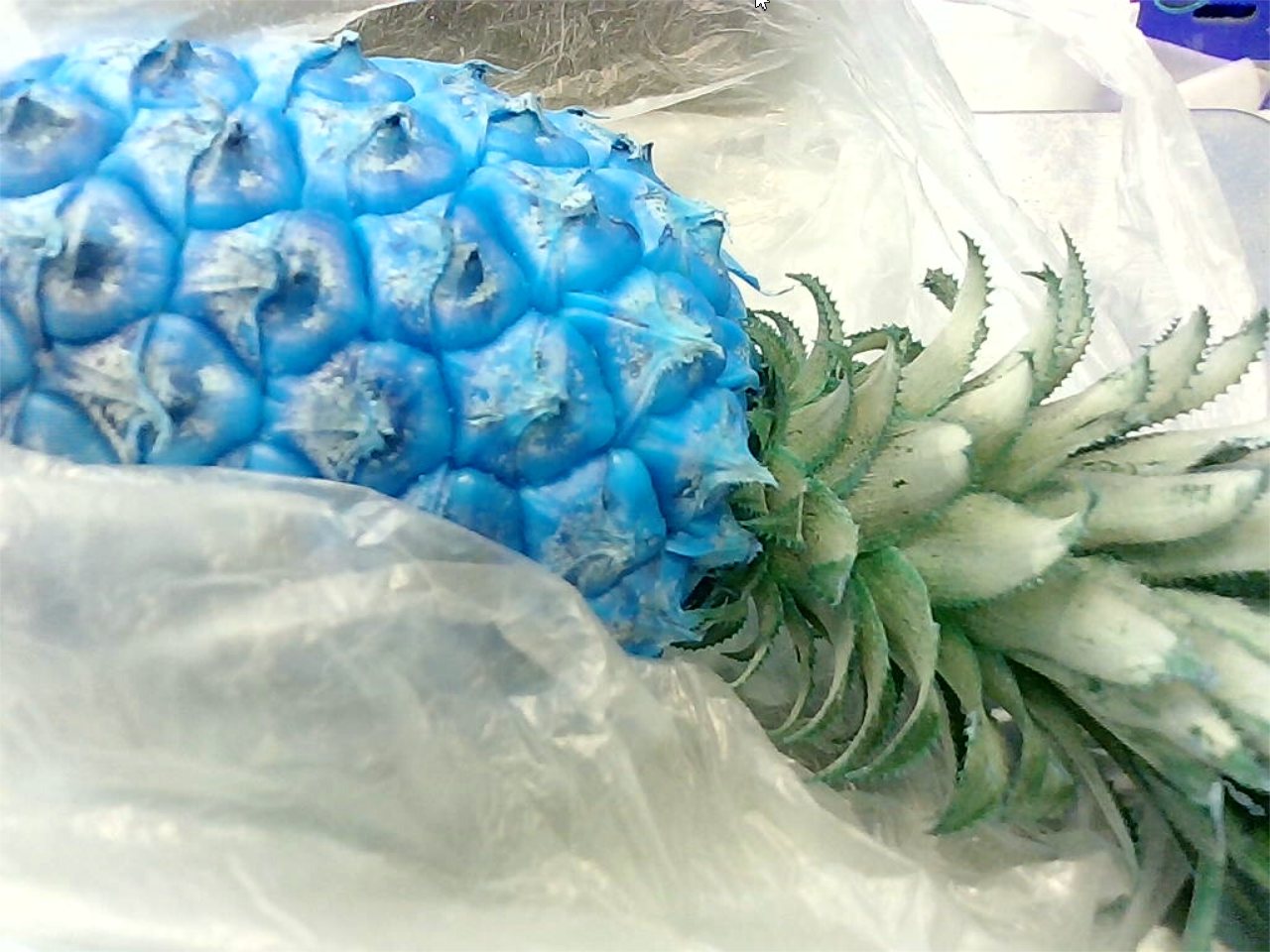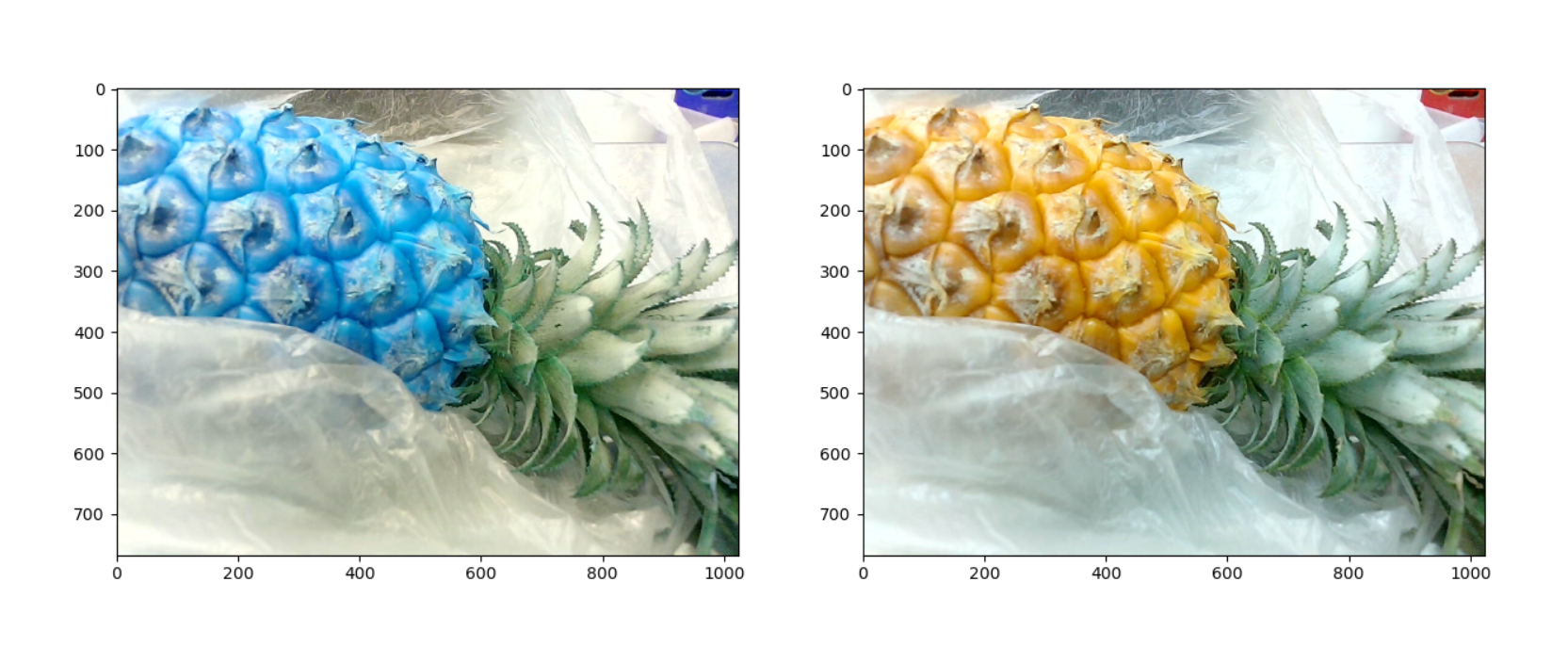# opencv读取图片通道以及显示

```cpp img=cv2.imread('515.jpg') cv2.imshow("aa",img) cv2.waitKey(-1)``` 显示结果：显示结果是正常的。如果转为RGB后？

```cpp import matplotlib.pyplot as plt import cv2

img=cv2.imread('515.jpg') img_rgb = cv2.cvtColor(img, cv2.COLOR_BGR2RGB) cv2.imshow("aa",img_rgb) cv2.waitKey(-1) ```然后却变成BGR了！为什么呢？难道cv2读取的通达不是BGR吗？ 我再换一种方式，使用matplotlib.pyplot显示图片。 代码：

```python img=cv2.imread('515.jpg') img_rgb = cv2.cvtColor(img, cv2.COLOR_BGR2RGB) fig, axes = plt.subplots(1, 2, figsize=(15, 15)) axes.imshow(img) axes.imshow(img_rgb) plt.show()``` 输出结果：结论：

cv2.imread( ) 所读取的图像格式：每个像素为[B,G,R]的形式 cv2.imshow( ) 显示图像：按图像中每个像素为[B,G,R]的规则将图像显示出来 所以使用cv2.imshow( )显示图像，我看到的是正常的图片。 如果换成matplotlib显示图像？ mpimg.imread( ) 所读取的图像格式：每个像素为[R,G,B]的形式 plt.imshow( ) 显示图像：按图像中每个像素为[R,G,B]的规则将图像显示出来 这时候图像就是偏蓝色的。 这也就是OpenCV读取的图片使用的时候，为啥要做BGR2RGB的转换。